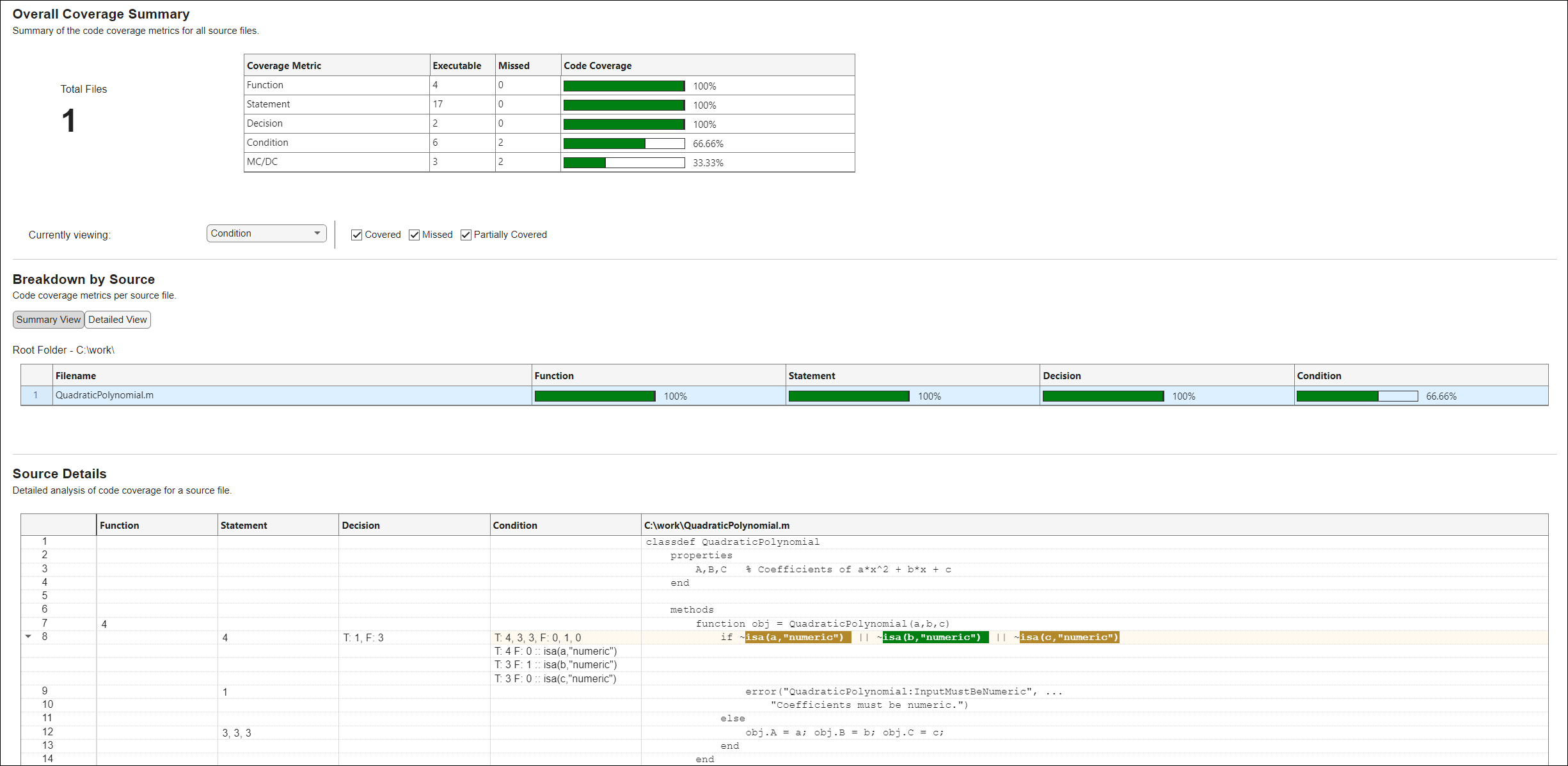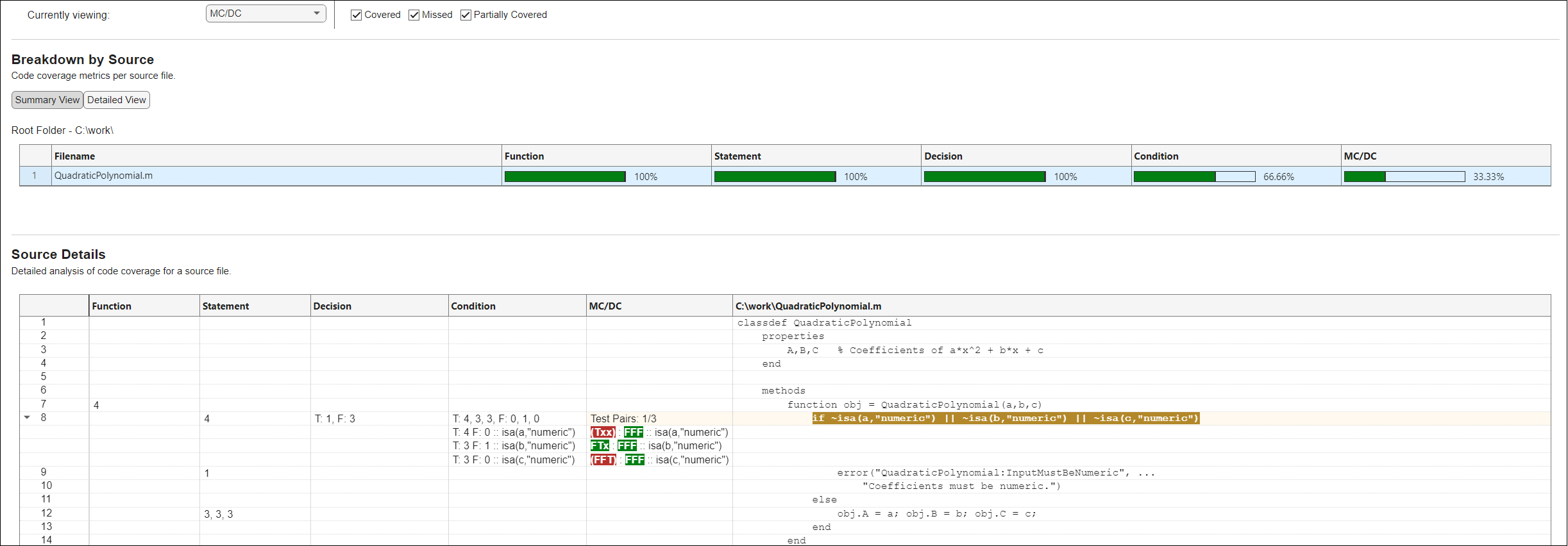# Collect Code Coverage Metrics for MATLAB Source Code

When you run tests, you can collect and access code coverage information for your MATLAB® source code by adding an instance of the `matlab.unittest.plugins.CodeCoveragePlugin` class to the test runner. The plugin supports several coverage types, including decision coverage, condition coverage, and modified condition/decision coverage (MC/DC). These coverage types let you perform a detailed analysis of the source code covered by the tests. For more information about coverage types, see Types of Code Coverage for MATLAB Source Code.

To perform a code coverage analysis using the supported coverage types, specify the `MetricLevel` name-value argument when you create a plugin using one of the static methods of the `CodeCoveragePlugin` class. The `MetricLevel` argument specifies which coverage types to include in the analysis. This list shows the possible values of `MetricLevel` and the included coverage types:

• `"statement"` (default value) — Statement and function coverage

• `"decision"` — Statement, function, and decision coverage

• `"condition"` — Statement, function, decision, and condition coverage

• `"mcdc"` — Statement, function, decision, condition, and modified condition/decision coverage

This example shows how to collect code coverage metrics and generate a report including all the supported coverage types for source code in a file. The file defines the `QuadraticPolynomial` class, which represents quadratic polynomials. The class constructor first validates that the coefficients of the polynomial are numeric values and then uses these values to initialize the class properties. The class includes the `solve` method to return the roots of the specified quadratic polynomial, and the `plot` method to plot the polynomial around its axis of symmetry. To view the complete code for `QuadraticPolynomial`, see `QuadraticPolynomial` Class Definition.

### Collect and Analyze Code Coverage Information

In your current folder, save the `QuadraticPolynomial` class definition in a file named `QuadraticPolynomial.m`. Then, create the `QuadraticPolynomialTest` test class in your current folder. The test class has four `Test` methods:

• `realSolution` — Test the `solve` method against a real solution.

• `imaginarySolution` — Test the `solve` method against an imaginary solution.

• `nonnumericInput` — Test the constructor method against a nonnumeric input.

• `plotPolynomial` — Test the `plot` method against a label.

```classdef QuadraticPolynomialTest < matlab.unittest.TestCase methods (Test) function realSolution(testCase) p = QuadraticPolynomial(1,-3,2); actSolution = p.solve(); expSolution = [1 2]; testCase.verifyEqual(actSolution,expSolution) end function imaginarySolution(testCase) p = QuadraticPolynomial(1,2,10); actSolution = p.solve(); expSolution = [-1-3i -1+3i]; testCase.verifyEqual(actSolution,expSolution) end function nonnumericInput(testCase) testCase.verifyError(@()QuadraticPolynomial(1,"-3",2), ... "QuadraticPolynomial:InputMustBeNumeric") end function plotPolynomial(testCase) p = QuadraticPolynomial(1,-3,2); fig = figure; testCase.addTeardown(@close,fig) ax = axes(fig); p.plot(ax) actYLabelText = ax.YLabel.String; expYLabelText = '1.00x^2-3.00x+2.00'; testCase.verifyEqual(actYLabelText,expYLabelText) end end end ```

To run tests and perform a code coverage analysis, first create a test runner with a plugin that provides programmatic access to information on all possible coverage types for the source code in the file `QuadraticPolynomial.m`.

```import matlab.unittest.plugins.CodeCoveragePlugin import matlab.unittest.plugins.codecoverage.CoverageResult runner = testrunner("textoutput"); format = CoverageResult; plugin = CodeCoveragePlugin.forFile("QuadraticPolynomial.m", ... Producing=format,MetricLevel="mcdc"); addPlugin(runner,plugin)```

Create a test suite from the `QuadraticPolynomialTest` class and run the tests. All the tests pass.

```suite = testsuite("QuadraticPolynomialTest"); run(runner,suite);```
```Running QuadraticPolynomialTest ... ```
```. Done QuadraticPolynomialTest __________ ```

After the test run, the `Result` property of the `CoverageResult` object holds the coverage result. You can use this result to programmatically access information about different coverage types. Additionally, you can generate a code coverage report from the result.

`result = format.Result;`

The `QuadraticPolynomial` class definition file has a single decision composed of three conditions. Access the decision coverage summary from the coverage result. The returned vector indicates that both the decision outcomes in the source code were achieved by the tests.

`decisionSummary = coverageSummary(result,"decision")`
```decisionSummary = 1×2 2 2 ```

Access the condition coverage summary. The summary indicates that the condition coverage is 66.7% because the tests missed two of the six possible condition outcomes in the `QuadraticPolynomial` class definition file.

`conditionSummary = coverageSummary(result,"condition")`
```conditionSummary = 1×2 4 6 ```

### Generate Code Coverage Report

Generate an HTML code coverage report from the coverage result. The report displays information about the collected code coverage information and uses different colors to highlight the executed or missed outcomes.

`generateHTMLReport(result)`

You can interact with the code coverage report. For example, in the Overall Coverage Summary section, you can select a coverage type from the Currently viewing list to view detailed information about that coverage type. The report has three sections:

• Overall Coverage Summary — This section displays the collected code coverage metrics for the source code.

• Breakdown by Source — This section displays the coverage metrics for each file in the source code. The value selected from the Currently viewing list determines which coverage metrics are displayed.

• Source Details — This section provides the analysis details for a file selected in the Breakdown by Source section. The value selected from the Currently viewing list determines the columns and code highlighting in the table.

For example, this figure shows the condition coverage view of the report. The Source Details section indicates that only the second condition in the source file was evaluated to both `true` and `false`. You can refer to the `Condition` column to learn how the tests evaluated each condition. For instance, `T: 4, 3, 3` shows that the tests evaluated the conditions to `true` for the specified number of times. On the other hand, `F: 0, 1, 0` shows that the second condition was evaluated to `false` a single time whereas the other two conditions were not evaluated to `false`. Out of six possible condition outcomes (that is, `true` and `false` for each of the three conditions), the tests achieved only four outcomes.Now, access the MC/DC view of the report by selecting `MC/DC` from the Currently viewing list. MC/DC identifies how tests independently exercise conditions within decisions. A condition receives MC/DC if tests can:

• Evaluate the condition to both `true` and `false`.

• Verify that the condition can independently affect the outcome of the decision it belongs to.

To examine these requirements, the testing framework finds the combinations of condition outcomes that tests must achieve for each condition. For example, this figure shows the Source Details section for the MC/DC type. The `MC/DC` column provides a pair of combinations for each condition, where `T`, `F`, and `x` denote `true`, `false`, and don't-care values, respectively. Achieving both combinations for a condition ensures that the condition evaluates to both `true` and `false` and that it independently affects the outcome of the decision:

• For the first condition to receive MC/DC, tests must achieve the `Txx` and `FFF` combinations.

• For the second condition to receive MC/DC, tests must achieve the `FTx` and `FFF` combinations.

• For the third condition to receive MC/DC, tests must achieve the `FFT` and `FFF` combinations.

Out of the `Txx`, `FFF`, `FTx`, and `FFT` combinations, the tests achieved only the `FFF` and `FTx` combinations. Therefore, only the second condition satisfied the MC/DC requirements.### `QuadraticPolynomial` Class Definition

This code provides the complete contents of the `QuadraticPolynomial` class.

```classdef QuadraticPolynomial properties A,B,C % Coefficients of a*x^2 + b*x + c end methods function obj = QuadraticPolynomial(a,b,c) if ~isa(a,"numeric") || ~isa(b,"numeric") || ~isa(c,"numeric") error("QuadraticPolynomial:InputMustBeNumeric", ... "Coefficients must be numeric.") else obj.A = a; obj.B = b; obj.C = c; end end function roots = solve(obj) % Return solutions to a*x^2 + b*x + c = 0 delta = calculateDelta(obj); roots(1) = (-obj.B - sqrt(delta)) / (2*obj.A); roots(2) = (-obj.B + sqrt(delta)) / (2*obj.A); end function plot(obj,ax) % Plot a*x^2 + b*x + c around its axis of symmetry delta = calculateDelta(obj); x0 = -obj.B/(2*obj.A); x1 = abs(sqrt(delta))/obj.A; x = x0 + linspace(-x1,x1); y = obj.A*x.^2 + obj.B*x + obj.C; plot(ax,x,y) xlabel("x") ylabel(sprintf("%.2fx^2%+.2fx%+.2f",obj.A,obj.B,obj.C)) end end methods (Access=private) function delta = calculateDelta(obj) delta = obj.B^2 - 4*obj.A*obj.C; end end end ```